# What is volatility¶

• Describes the dispersion of financial asset returns over time.
• Often computed as the standard deviation or variance of price returns.
• The higher the volatility, the riskier a financial asset.

## The challenge of volatility modeling¶

Heteroskedasticity:

• In ancient Greek: "different" (hetero) + "dispersion" (skedasis)
• A time series demonstrates varying volatility systematically over time.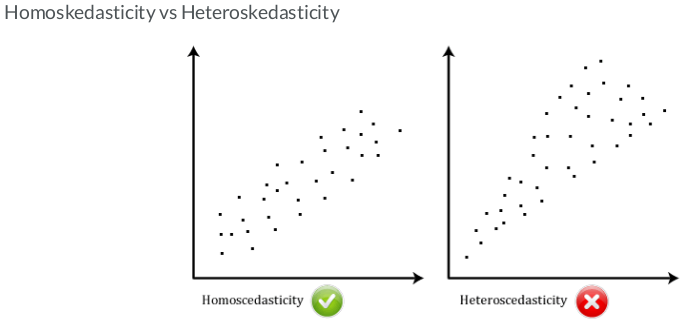## Imports & Settings¶

In :
import datetime as dt
import sys
import numpy as np
from numpy import cumsum, log, polyfit, sqrt, std, subtract
from numpy.random import randn
import pandas as pd
from pandas_datareader import data as web
import seaborn as sns
from pylab import rcParams
import matplotlib.pyplot as plt
import matplotlib.cm as cm
from arch import arch_model
from numpy.linalg import LinAlgError
from scipy import stats
import statsmodels.api as sm
import statsmodels.tsa.api as tsa
from statsmodels.graphics.tsaplots import plot_acf, plot_pacf
from statsmodels.tsa.stattools import acf, q_stat, adfuller
from sklearn.metrics import mean_squared_error
from scipy.stats import probplot, moment
from arch import arch_model
from arch.univariate import ConstantMean, GARCH, Normal
from sklearn.model_selection import TimeSeriesSplit
import warnings

In :
%matplotlib inline
pd.set_option('display.max_columns', None)
warnings.filterwarnings('ignore')
sns.set(style="darkgrid", color_codes=True)
rcParams['figure.figsize'] = 8,4


# Hurst Exponent function¶

The Hurst Exponent is a statistical measure used to classify time series and infer the level of difficulty in predicting and choosing an appropriate model for the series at hand. The Hurst exponent is used as a measure of long-term memory of time series. It relates to the autocorrelations of the time series, and the rate at which these decrease as the lag between pairs of values increases.

• Value near 0.5 indicates a random series.
• Value near 0 indicates a mean reverting series.
• Value near 1 indicates a trending series.
In :
def hurst(ts):
"""Returns the Hurst Exponent of the time series vector ts"""
# Create the range of lag values
lags = range(2, 100)

# Calculate the array of the variances of the lagged differences
tau = [sqrt(std(subtract(ts[lag:], ts[:-lag]))) for lag in lags]

# Use a linear fit to estimate the Hurst Exponent
poly = polyfit(log(lags), log(tau), 1)

# Return the Hurst exponent from the polyfit output
return poly*2.0


## Correlogram Plot¶

In :
def plot_correlogram(x, lags=None, title=None):
lags = min(10, int(len(x)/5)) if lags is None else lags
fig, axes = plt.subplots(nrows=2, ncols=2, figsize=(12, 8))
x.plot(ax=axes)
q_p = np.max(q_stat(acf(x, nlags=lags), len(x)))
axes.text(x=.02, y=.85, s=stats, transform=axes.transAxes)
probplot(x, plot=axes)
mean, var, skew, kurtosis = moment(x, moment=[1, 2, 3, 4])
s = f'Mean: {mean:>12.2f}\nSD: {np.sqrt(var):>16.2f}\nSkew: {skew:12.2f}\nKurtosis:{kurtosis:9.2f}'
axes.text(x=.02, y=.75, s=s, transform=axes.transAxes)
plot_acf(x=x, lags=lags, zero=False, ax=axes)
plot_pacf(x, lags=lags, zero=False, ax=axes)
axes.set_xlabel('Lag')
axes.set_xlabel('Lag')
fig.suptitle(title, fontsize=20)
fig.tight_layout()


We will use daily S&P 500 returns from 2005-2020 to demonstrate the usage of a GARCH model

In :
start = pd.Timestamp('2005-01-01')
end = pd.Timestamp('2020-04-09')

sp_data = web.DataReader('SPY', 'yahoo', start, end)\

sp_data.info()

<class 'pandas.core.frame.DataFrame'>
DatetimeIndex: 3844 entries, 2005-01-03 to 2020-04-09
Data columns (total 6 columns):
High         3844 non-null float64
Low          3844 non-null float64
Open         3844 non-null float64
Close        3844 non-null float64
Volume       3844 non-null float64
dtypes: float64(6)
memory usage: 210.2 KB

In :
sp_data.head()

Out:
High Low Open Close Volume Adj Close
Date
2005-01-03 121.760002 119.900002 121.559998 120.300003 55748000.0 88.533607
2005-01-04 120.540001 118.440002 120.459999 118.830002 69167600.0 87.451752
2005-01-05 119.250000 118.000000 118.739998 118.010002 65667300.0 86.848251
2005-01-06 119.150002 118.260002 118.440002 118.610001 47814700.0 87.289810
2005-01-07 119.230003 118.129997 118.970001 118.440002 55847700.0 87.164726

## Observe volatility clustering¶

Volatility clustering refers to the observation that "large changes tend to be followed by large changes, of either sign, and small changes tend to be followed by small changes.

• Volatility clustering is frequently observed in financial market data, and it poses a challenge for time series modeling.

with the S&P 500 daily price dataset we calculate daily returns as the percentage price changes, plot the results and observe its behavior over time.

In :
# Calculate daily returns as percentage price changes
sp_data['Return'] = 100 * (sp_data['Close'].pct_change())
sp_data['Log_Return'] = np.log(sp_data['Close']).diff().mul(100) # rescale to faciliate optimization
sp_data = sp_data.dropna()

# Plot ACF, PACF and Q-Q plot and get ADF p-value of series
plot_correlogram(sp_data['Log_Return'], lags=100, title='S&P 500 (Log, Diff)')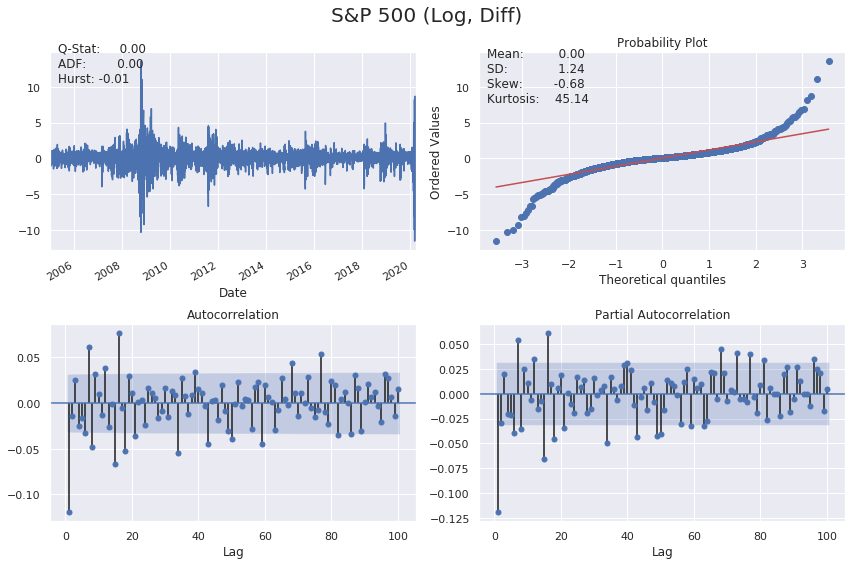In :
plot_correlogram(sp_data['Log_Return'].sub(sp_data['Log_Return'].mean()).pow(2), lags=100, title='S&P 500 Daily Volatility')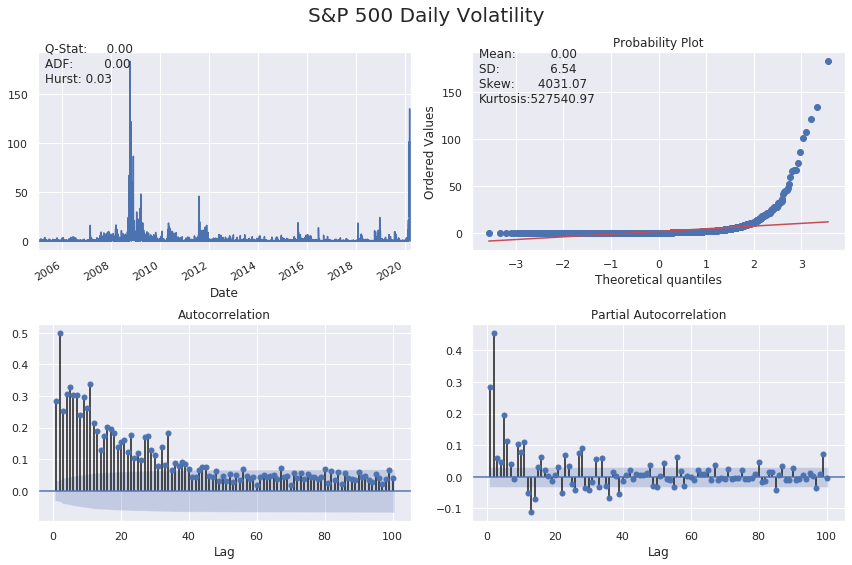## Calculate volatility¶

We compute and convert volatility of price returns in Python.

Firstly, we compute the daily volatility as the standard deviation of price returns. Then convert the daily volatility to monthly and annual volatility.

In :
# Calculate daily std of returns
std_daily = sp_data['Return'].std()
print(f'Daily volatility: {round(std_daily,2)}%')

# Convert daily volatility to monthly volatility
std_monthly = np.sqrt(21) * std_daily
print(f'\nMonthly volatility: {round(std_monthly,2)}%')

# Convert daily volatility to annaul volatility
std_annual = np.sqrt(252) * std_daily
print(f'\nAnnual volatility: {round(std_annual,2)}%')

Daily volatility: 1.24%

Monthly volatility: 5.67%

Annual volatility: 19.63%


# ARCH and GARCH¶

First came the ARCH

• Auto Regressive Conditional Heteroskedasticity
• Developed by Robert F. Engle (Nobel prize laureate 2003)

Then came the GARCH

• "Generalized" ARCH
• Developed by Tim Bollerslev (Robert F. Engle's student)

## Model notations¶

• Expected return: $$\mu = Expected|r_t|I(t-1)$$

• Expected volatility: $$\sigma^2 = Expected[(r_t - \mu_t)^2|I(t - 1)]$$

• Residual (prediction error): $$r_t = \mu + \epsilon_t$$

• Volatility is related to the residuals: $$\epsilon_t = \sigma_t * \zeta(WhiteNoise)$$

• White noise (z): Uncorrelated random variables with a zero mean and a finite variance

## Model intuition¶

• Autoregressive: predict future behavior based on past behavior.
• Volatility as a weighted average of past information.

### GARCH(1,1) parameter constraints¶

• All parameters are non-negative, so the variance cannot be negative. $$\omega, \alpha, \beta >= 0$$

• Model estimations are "mean-reverting" to the long-run variance. $$\alpha + \beta < 1$$

• long-run variance: $$\omega \: / \: (1 - \alpha - \beta)$$

### GARCH(1,1) parameter dynamics¶

• The larger the α , the bigger the immediate impact of the shock
• The larger the β , the longer the duration of the impact

Given the GARCH(1,1) model equation as:

$$GARCH(1,1): \sigma_t^2 = \omega + \alpha \epsilon_{t-1}^2 + \beta \sigma_{t-1}^2$$

Intuitively, GARCH variance forecast can be interpreted as a weighted average of three different variance forecasts.

• One is a constant variance that corresponds to the long run average.
• The second is the new information that was not available when the previous forecast was made.
• The third is the forecast that was made in the previous period.

The weights on these three forecasts determine how fast the variance changes with new information and how fast it reverts to its long run mean.

# Simulate ARCH and GARCH series¶

We will simulate an ARCH(1) and GARCH(1,1) time series respectively using a function simulate_GARCH(n, omega, alpha, beta = 0).

Recall the difference between an ARCH(1) and a GARCH(1,1) model is: besides an autoregressive component of α multiplying lag-1 residual squared, a GARCH model includes a moving average component of β multiplying lag-1 variance.

The function will simulate an ARCH/GARCH series based on n (number of simulations), omega, alpha, and beta (0 by default) you specify. It will return simulated residuals and variances.

In :
def simulate_GARCH(n, omega, alpha, beta = 0):
np.random.seed(4)
# Initialize the parameters
white_noise = np.random.normal(size = n)
resid = np.zeros_like(white_noise)
variance = np.zeros_like(white_noise)

for t in range(1, n):
# Simulate the variance (sigma squared)
variance[t] = omega + alpha * resid[t-1]**2 + beta * variance[t-1]
# Simulate the residuals
resid[t] = np.sqrt(variance[t]) * white_noise[t]

return resid, variance

In :
# Simulate a ARCH(1) series
arch_resid, arch_variance = simulate_GARCH(n= 200,
omega = 0.1, alpha = 0.7)
# Simulate a GARCH(1,1) series
garch_resid, garch_variance = simulate_GARCH(n= 200,
omega = 0.1, alpha = 0.7,
beta = 0.1)

# Plot the ARCH variance
plt.figure(figsize=(10,5))
plt.plot(arch_variance, color = 'red', label = 'ARCH Variance')

# Plot the GARCH variance
plt.plot(garch_variance, color = 'orange', label = 'GARCH Variance')
plt.legend()
plt.show()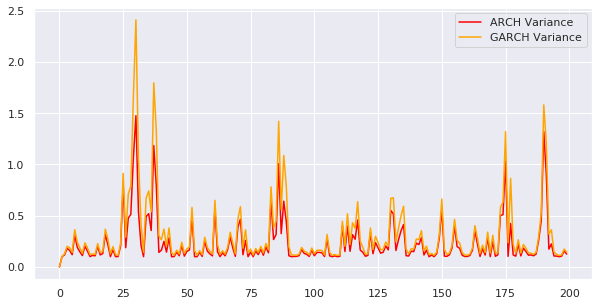## Observe the impact of model parameters¶

We will call the function simulate_GARCH() again, and study the impact of GARCH model parameters on simulated results.

Specifically, we will simulate two GARCH(1,1) time series, they have the same omega and alpha, but different beta as input.

Recall in GARCH(1,1), since β is the coefficient of lag-1 variance, if the α is fixed, the larger the β, the longer the duration of the impact. In other words, high or low volatility periods tend to persist. Pay attention to the plotted results and see whether we can verify the β impact.

In :
# First simulated GARCH
plt.figure(figsize=(10,3))
sim_resid, sim_variance = simulate_GARCH(n = 200,  omega = 0.1, alpha = 0.3, beta = 0.2)
plt.plot(sim_variance, color = 'orange', label = 'Variance')
plt.plot(sim_resid, color = 'green', label = 'Residuals')
plt.title('First simulated GARCH, Beta = 0.2')
plt.legend(loc='best')
plt.show()

# Second simulated GARCH
plt.figure(figsize=(10,3))
sim_resid, sim_variance = simulate_GARCH(n = 200,  omega = 0.1, alpha = 0.3, beta = 0.6)
plt.plot(sim_variance, color = 'red', label = 'Variance')
plt.plot(sim_resid, color = 'deepskyblue', label = 'Residuals')
plt.title('Second simulated GARCH, Beta = 0.6')
plt.legend(loc='best')
plt.show()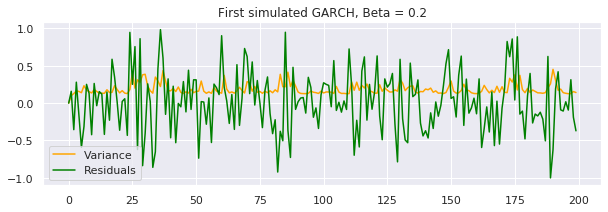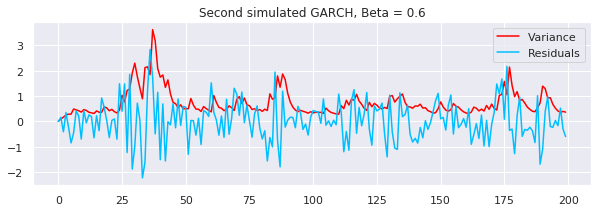# Implement a basic GARCH model¶

We will get familiar with the Python arch package, and use its functions such as arch_model() to implement a GARCH(1,1) model.

First define a basic GARCH(1,1) model, then fit the model, review the model fitting summary, and plot the results.

In :
# Specify GARCH model assumptions
basic_gm = arch_model(sp_data['Return'], p = 1, q = 1,
mean = 'constant', vol = 'GARCH', dist = 'normal')
# Fit the model
gm_result = basic_gm.fit(update_freq = 4)

Iteration:      4,   Func. Count:     36,   Neg. LLF: 4997.204823442513
Iteration:      8,   Func. Count:     64,   Neg. LLF: 4993.358477465824
Iteration:     12,   Func. Count:     88,   Neg. LLF: 4992.874678320535
Optimization terminated successfully.    (Exit mode 0)
Current function value: 4992.874653095375
Iterations: 13
Function evaluations: 94

In :
# Display model fitting summary
print(gm_result.summary())

                     Constant Mean - GARCH Model Results
==============================================================================
Dep. Variable:                 Return   R-squared:                      -0.001
Mean Model:             Constant Mean   Adj. R-squared:                 -0.001
Vol Model:                      GARCH   Log-Likelihood:               -4992.87
Distribution:                  Normal   AIC:                           9993.75
Method:            Maximum Likelihood   BIC:                           10018.8
No. Observations:                 3843
Date:                Mon, Apr 13 2020   Df Residuals:                     3839
Time:                        20:55:47   Df Model:                            4
Mean Model
============================================================================
coef    std err          t      P>|t|      95.0% Conf. Int.
----------------------------------------------------------------------------
mu             0.0720  1.188e-02      6.062  1.343e-09 [4.873e-02,9.529e-02]
Volatility Model
============================================================================
coef    std err          t      P>|t|      95.0% Conf. Int.
----------------------------------------------------------------------------
omega          0.0268  5.823e-03      4.603  4.172e-06 [1.539e-02,3.821e-02]
alpha       0.1414  1.592e-02      8.885  6.370e-19     [  0.110,  0.173]
beta        0.8372  1.540e-02     54.376      0.000     [  0.807,  0.867]
============================================================================

Covariance estimator: robust

In :
# Plot fitted results
gm_result.plot()
plt.show()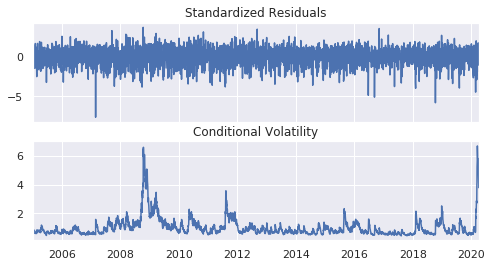### Make forecast with GARCH models¶

We will practice making a basic volatility forecast.

We will call .forecast() to make a prediction. By default it produces a 1-step ahead estimate. You can use horizon = n to specify longer forward periods.

In :
# Make 5-period ahead forecast
gm_forecast = gm_result.forecast(horizon = 5)

# Print the forecast variance
print(gm_forecast.variance[-1:])

                  h.1        h.2        h.3        h.4        h.5
Date
2020-04-09  12.396423  12.157934  11.924549  11.696159  11.472656


h.1 in row "2020-04-09": is a 1-step ahead forecast made using data up to and including that date

# Distribution assumptions¶

Why make assumptions?

• Volatility is not directly observable
• GARCH model use residuals as volatility shocks $$r_t = \mu + t + \epsilon_t$$
• Volatility is related to the residuals: $$\epsilon_t = \sigma_t * \zeta(WhiteNoise)$$

Standardized residuals

• Residual = predicted return - mean return $$residuals = \epsilon_t = r_t - \mu_t$$
• Standardized residual = residual / return volatility $$std Resid = \frac{\epsilon_t}{\sigma_t}$$

GARCH models make distribution assumptions about the residuals and the mean return. Financial time series data often does not follow a normal distribution. In financial time series it is much more likely to observe extreme positive and negative values that are far away from the mean. to improve a GARCH models distribution assumptions to be more representative of real financial data we can specify the models distribution assumption to be a Student's t-distribution. A Student's t-distribution is symmetric and bell shaped similar to a normal distribution but has fatter tails making it more prone to producing values that fall far away from its mean. The nu $(\nu)$ parameter indicates its shape the larger the $\nu$ the more peaked the curve becomes.

GARCH models enable one to specify the distribution assumptions of the standardized residuals. By default, a normal distribution is assumed, which has a symmetric, bell-shaped probability density curve. Other options include Student's t-distribution and skewed Student's t-distribution.

### Plot distribution of standardized residuals¶

We will practice computing the standardized residuals from a fitted GARCH model, and then plot its histogram together with a normal fit distribution normal_resid.

In :
# Obtain model estimated residuals and volatility
gm_resid = gm_result.resid
gm_std = gm_result.conditional_volatility

# Calculate the standardized residuals
gm_std_resid = gm_resid /gm_std

# Plot the histogram of the standardized residuals
plt.figure(figsize=(7,4))
sns.distplot(gm_std_resid, norm_hist=True, fit=stats.norm, bins=50, color='r')
plt.legend(('normal', 'standardized residuals'))
plt.show()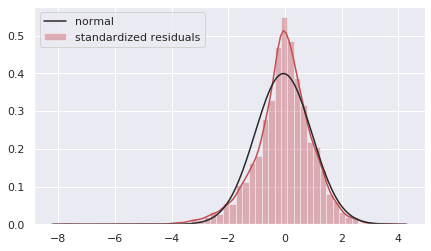# Fit a GARCH with skewed t-distribution¶

We will improve the GARCH model by using a skewed Student's t-distribution assumption. In addition.

In :
# Specify GARCH model assumptions
skewt_gm = arch_model(sp_data['Return'], p = 1, q = 1, mean = 'constant', vol = 'GARCH', dist = 'skewt')

# Fit the model
skewt_result = skewt_gm.fit(disp = 'off')

# Get model estimated volatility
skewt_vol = skewt_result.conditional_volatility

In :
# Plot model fitting results
plt.plot(skewt_vol, color = 'red', label = 'Skewed-t Volatility')
plt.plot(sp_data['Return'], color = 'grey',
label = 'Daily Returns', alpha = 0.4)
plt.legend(loc = 'upper right')
plt.show()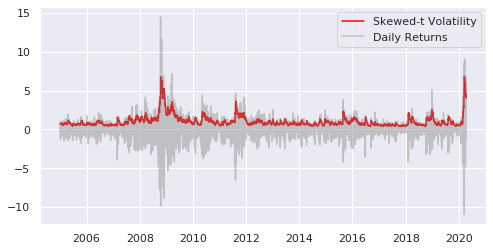## Mean model specifications¶

• Constant mean: generally works well with most financial return data.

• Autoregressive mean: model the mean as an autoregressive (AR) process.

• Zero mean: Use when the mean has been modeled separately to fit the residuals of the separate model to estimate volatility (preferred method).

Here we model the log-returns of the S&P 500 data with an ARMA model and then fit the models residuals to estimate the volatility of the returns series with a GARCHmodel.

Searching over model orders to find the optimal number of lags

In :
import pmdarima as pm

model = pm.auto_arima(sp_data['Log_Return'],

d=0, # non-seasonal difference order
start_p=1, # initial guess for p
start_q=1, # initial guess for q
max_p=4, # max value of p to test
max_q=4, # max value of q to test

seasonal=False, # is the time series seasonal

information_criterion='bic', # used to select best model
trace=True, # print results whilst training
error_action='ignore', # ignore orders that don't work
stepwise=True, # apply intelligent order search

)

Performing stepwise search to minimize bic
Fit ARIMA: (1, 0, 1)x(0, 0, 0, 0) (constant=True); AIC=12497.795, BIC=12522.811, Time=0.288 seconds
Fit ARIMA: (0, 0, 0)x(0, 0, 0, 0) (constant=True); AIC=12551.752, BIC=12564.260, Time=0.062 seconds
Fit ARIMA: (1, 0, 0)x(0, 0, 0, 0) (constant=True); AIC=12498.379, BIC=12517.141, Time=0.110 seconds
Fit ARIMA: (0, 0, 1)x(0, 0, 0, 0) (constant=True); AIC=12496.119, BIC=12514.881, Time=0.185 seconds
Fit ARIMA: (0, 0, 0)x(0, 0, 0, 0) (constant=False); AIC=12550.945, BIC=12557.199, Time=0.037 seconds
Fit ARIMA: (0, 0, 2)x(0, 0, 0, 0) (constant=True); AIC=12497.705, BIC=12522.721, Time=0.453 seconds
Fit ARIMA: (1, 0, 2)x(0, 0, 0, 0) (constant=True); AIC=12493.967, BIC=12525.237, Time=1.152 seconds
Total fit time: 2.290 seconds

In :
print(model.summary())

                               SARIMAX Results
==============================================================================
Dep. Variable:                      y   No. Observations:                 3843
Model:               SARIMAX(0, 0, 1)   Log Likelihood               -6245.060
Date:                Mon, 13 Apr 2020   AIC                          12496.119
Time:                        20:55:59   BIC                          12514.881
Sample:                             0   HQIC                         12502.783
- 3843
Covariance Type:                  opg
==============================================================================
coef    std err          z      P>|z|      [0.025      0.975]
------------------------------------------------------------------------------
intercept      0.0218      0.018      1.180      0.238      -0.014       0.058
ma.L1         -0.1242      0.008    -16.509      0.000      -0.139      -0.109
sigma2         1.5101      0.012    123.107      0.000       1.486       1.534
===================================================================================
Ljung-Box (Q):                      115.36   Jarque-Bera (JB):             38226.99
Prob(Q):                              0.00   Prob(JB):                         0.00
Heteroskedasticity (H):               0.58   Skew:                            -0.58
Prob(H) (two-sided):                  0.00   Kurtosis:                        18.41
===================================================================================

Warnings:
 Covariance matrix calculated using the outer product of gradients (complex-step).

In :
# Fit best model
_arma_model = sm.tsa.SARIMAX(endog=sp_data['Log_Return'],order=(0, 0, 1))
_model_result = _arma_model.fit()

In :
# Plot model residuals
_model_result.plot_diagnostics(figsize=(10, 5))
plt.show()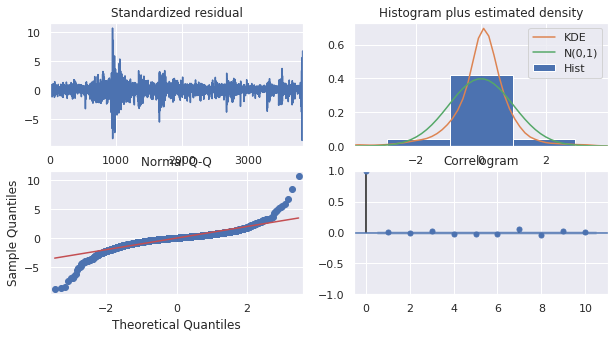In :
# Fit GARCH model with ARMA model residuals
_garch_model = arch_model(_model_result.resid, mean='Zero', p=1, q=1)
_garch_result = _garch_model.fit(disp = 'off')
print(_garch_result.summary())

                       Zero Mean - GARCH Model Results
==============================================================================
Dep. Variable:                   None   R-squared:                       0.000
Mean Model:                 Zero Mean   Adj. R-squared:                  0.000
Vol Model:                      GARCH   Log-Likelihood:               -5020.26
Distribution:                  Normal   AIC:                           10046.5
Method:            Maximum Likelihood   BIC:                           10065.3
No. Observations:                 3843
Date:                Mon, Apr 13 2020   Df Residuals:                     3840
Time:                        20:56:02   Df Model:                            3
Volatility Model
============================================================================
coef    std err          t      P>|t|      95.0% Conf. Int.
----------------------------------------------------------------------------
omega          0.0258  5.748e-03      4.481  7.443e-06 [1.449e-02,3.702e-02]
alpha       0.1319  1.524e-02      8.650  5.169e-18     [  0.102,  0.162]
beta        0.8467  1.526e-02     55.488      0.000     [  0.817,  0.877]
============================================================================

Covariance estimator: robust

In :
# Plot GARCH model fitted results
_garch_result.plot()
plt.show()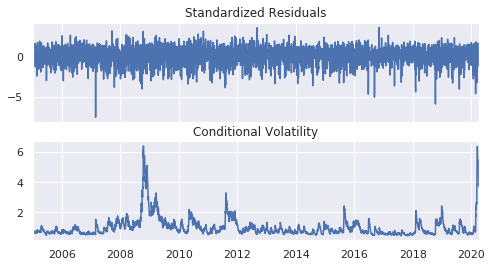In :
plot_correlogram(_garch_result.resid.dropna(), lags=100, title='GARCH Residuals')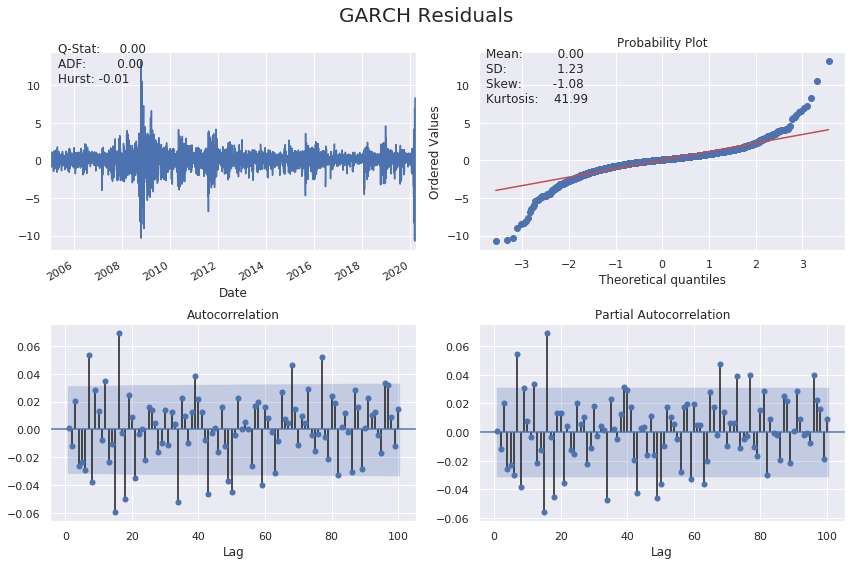# Modeling of asymmetric responses of volatility¶

Leverage effect

• Debt-equity Ratio = Debt / Equity
• Stock price goes down, debt-equity ratio goes up
• Riskier!

GARCH models assume positive and negative news has a symmetric impact on volatility. However, in reality the market tends to take the stairs up and the elevator down. In other words, the impact is usually asymmetric, and negative news tends to affect the volatility more than positive news.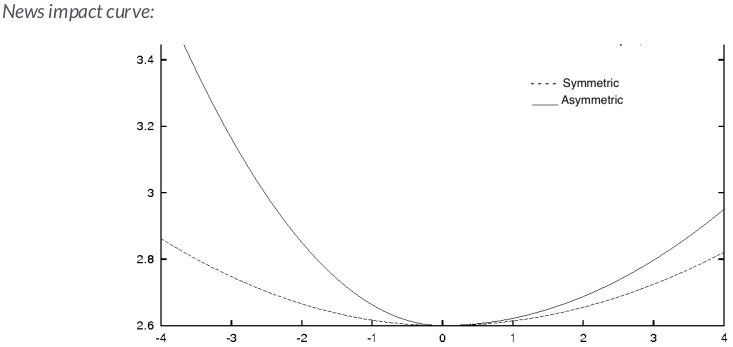GARCH models that account for asymmetric shocks:

• GJR-GARCH
• A popular option to model asymmetric shocks
• GJR-GARCH in Python: arch_model(my_data, p = 1, q = 1, o = 1, mean = 'constant', vol = 'GARCH')

GJR-GARCH:

$$\sigma_t^2 = \omega + (\alpha + \gamma I_{t-1} + \beta \sigma_{t-1}^2$$

$$I_{t-1} := \begin{cases} 0 \text{ if } r_{t-1} \geq \mu \\ 1 \text{ if } r_{t-1} < \mu \end{cases}$$

• EGARCH
• Exponential GARCH
• Add a conditional component to model the asymmetry in shocks similar to the GJR-GARCH
• No non-negative constraints on alpha, beta so it runs faster
• GJR-GARCH in Python: arch_model(my_data, p = 1, q = 1, o = 1, mean = 'constant', vol = EGARCH)

EGARCH:

$$log \: \sigma_t^2 = \omega + \sum_{k=1}^q \beta_k g (Z_t - k) + \sum_{k=1}^p \alpha_k \: log \: \sigma_{t-k}^2$$

where $g(Z_t) = \theta Z_t + \lambda(|Z_t| - E(|Z_t|)), \sigma_t^2$ is the conditional variance, $\omega,\beta, \alpha, \theta \text{ and } \lambda$ are coefficients.

# Fit GARCH models to cryptocurrency¶

Financial markets tend to react to positive and negative news shocks very differently, and one example is the dramatic swings observed in the cryptocurrency market in recent years.

We will implement a GJR-GARCH and an EGARCH model respectively in Python, which are popular choices to model the asymmetric responses of volatility.

Load the daily Bitcoin price from the Blockchain.com API:

In :
bitcoin_data = pd.read_csv('https://api.blockchain.info/charts/market-price?start=2010-10-09&timespan=12years&format=csv',
names=['Timestamp','Close'], index_col='Timestamp')
bitcoin_data.index = pd.to_datetime(bitcoin_data.index, format='%Y-%m-%d')
bitcoin_data = bitcoin_data.loc[(bitcoin_data != 0.0).any(axis=1)]
bitcoin_data['Return'] = np.log(bitcoin_data['Close']).diff().mul(100) # rescale to faciliate optimization
bitcoin_data = bitcoin_data.dropna()
bitcoin_data.info()

<class 'pandas.core.frame.DataFrame'>
DatetimeIndex: 1737 entries, 2010-10-11 to 2020-04-13
Data columns (total 2 columns):
Close     1737 non-null float64
Return    1737 non-null float64
dtypes: float64(2)
memory usage: 40.7 KB

In :
# Plot bitcoin price data
fig, ax1 = plt.subplots(figsize=(13, 5))
ax1.set_yscale('log')
ax1.plot(bitcoin_data.index, bitcoin_data.Close, color='b', label='BTCUSD')
ax1.set_xlabel('Date')
ax1.set_ylabel('BTCUSD Close')
ax1.legend()
plt.show()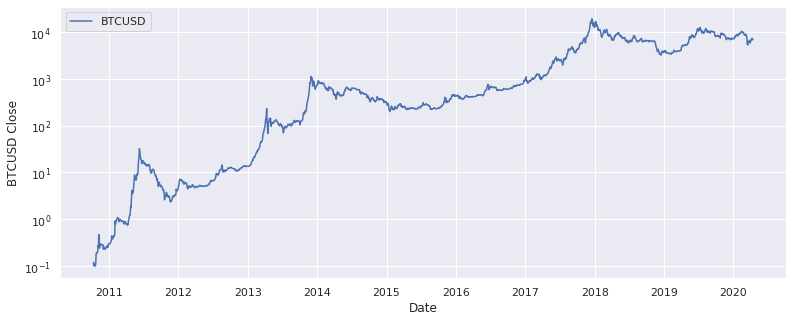In :
plot_correlogram(bitcoin_data['Return'], lags=100, title='BTCUSD (Log, Diff)')